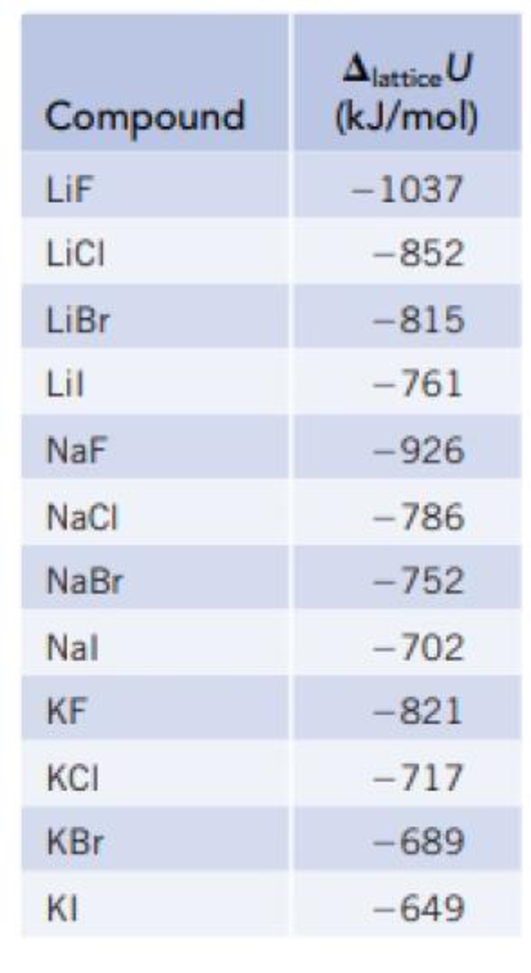Chapter 12, Problem 17PS

Chapter
Section
Textbook Problem

Calculate the molar enthalpy of formation, ΔfH°, of solid lithium fluoride from the lattice energy (Table 12.1) and other thermochemical data. The enthalpy of formation of Li(g), ΔfH° [Li(g)] = 159.37 kJ/mol. Other required data can be found in Appendices F and L.TABLE 12.1Lattice Energies of Some Ionic CompoundsInterpretation Introduction

Interpretation:

The molar enthalpy formation of lithium fluoride has to be calculated.

Concept introduction:

The molar enthalpy formation is calculated by using following method,

ΔfHo=ΔsubHo+IE+Do+EA+ΔlatticeU

Explanation

The molar enthalpy formation is calculated by using Hess law, the enthalpy of the formation is given below

ΔfHo=ΔsubHo+IE+Do+EA+ΔlatticeUΔsubHo=+159.37kJ/molIE=+520kJ/molDo=+78

Still sussing out bartleby?

Check out a sample textbook solution.

See a sample solution

The Solution to Your Study Problems

Bartleby provides explanations to thousands of textbook problems written by our experts, many with advanced degrees!

Get Started